# Water Quality Module

With this compact device, all you have to do to acquire the water quality information of any water source is drop it in!## Things used in this project

### Hardware components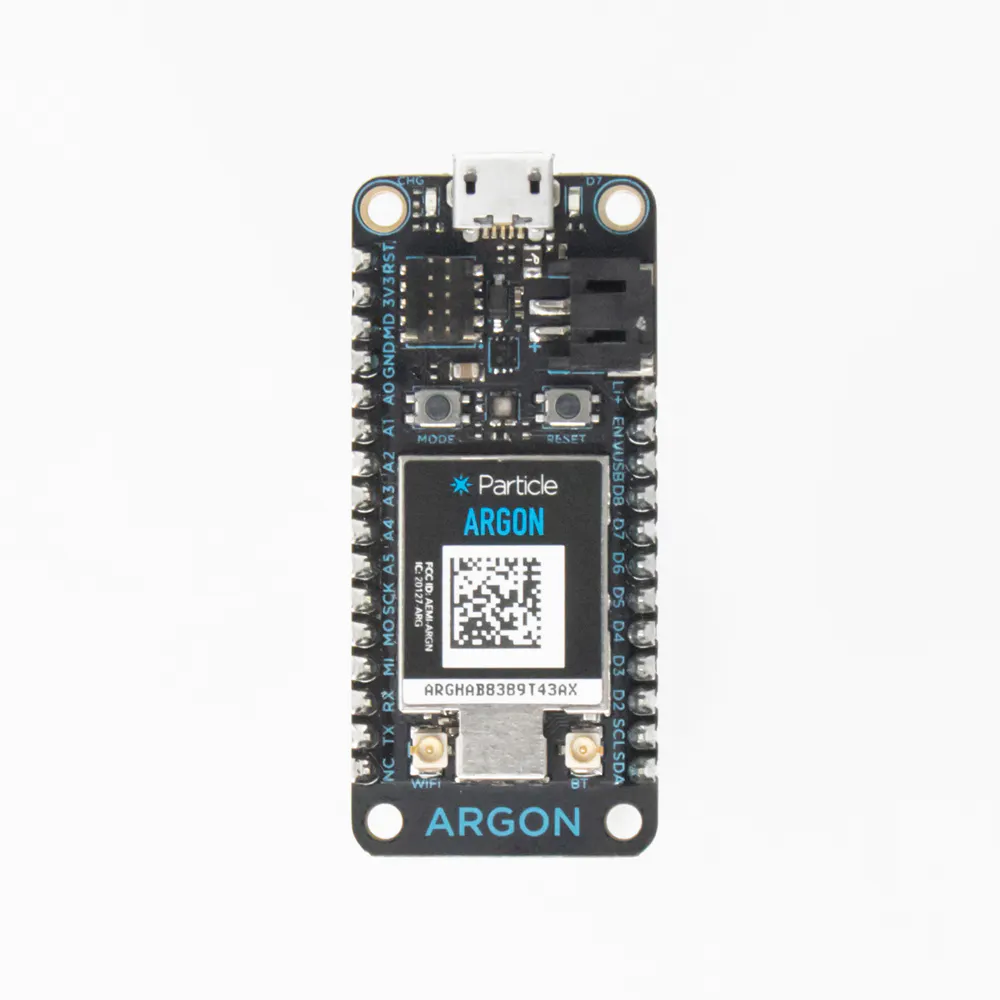Particle Argon
×1
 Waterproof Temperature Sensor
×1
 pH Sensor
×1
 TDS Meter Sensor
×1

### Software apps and online servicesParticle Build Web IDE

### Hand tools and fabrication machines

 Creality CR-10s 3D Printer

## Custom parts and enclosures

### Enclosure V2

This box will keep the electrical components safe from any exposure to water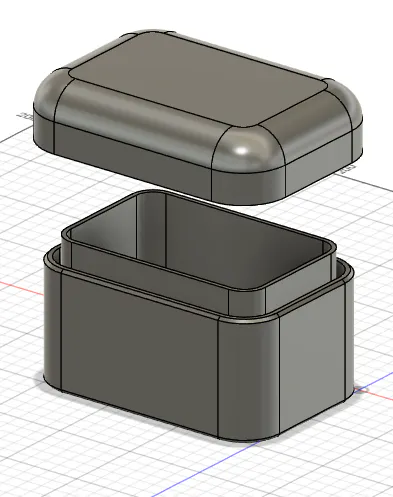## Schematics

### Temperature Sensor Schematic

This is the wiring setup for the Temperature Sensor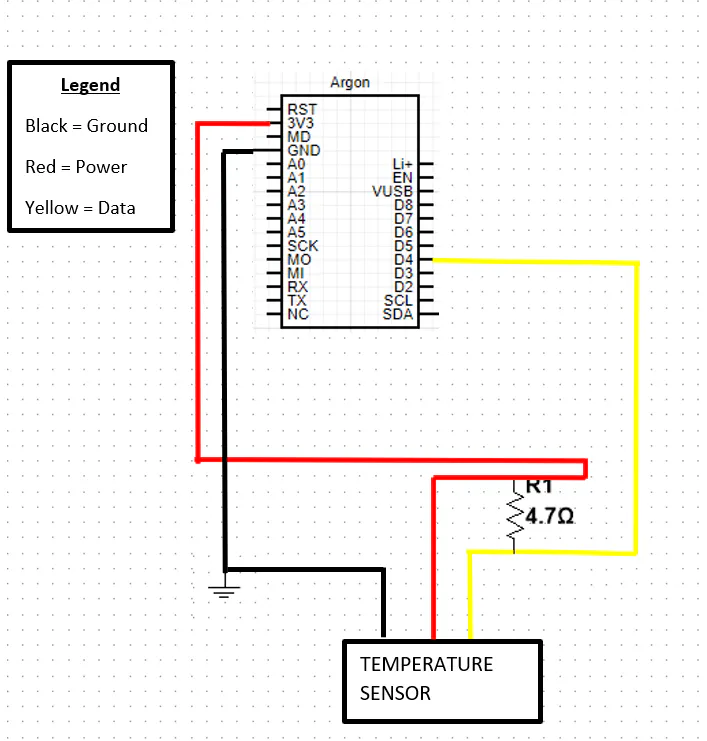### PH Sensor Schematic

This is the wiring for the PH Sensor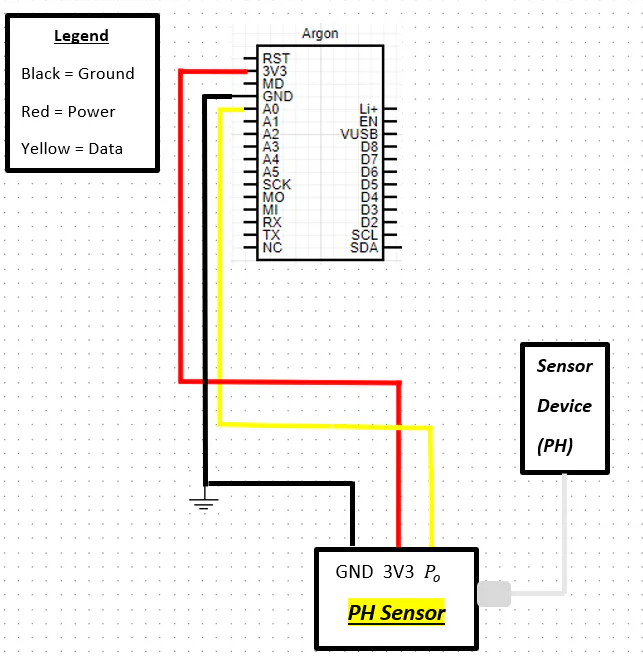### Communcation Diagram

This Diagram depicts the Water Quality Sensor and how it communicates with a mobile device.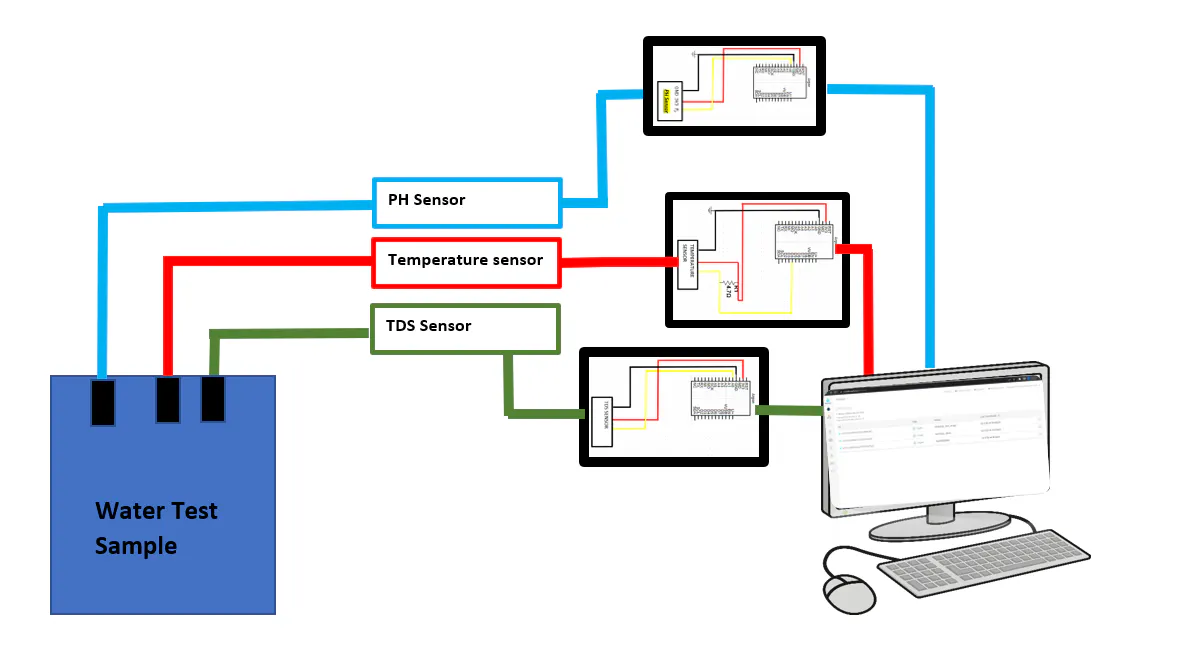### TDS Sensor Circuit

This is the wiring for the TDS sensor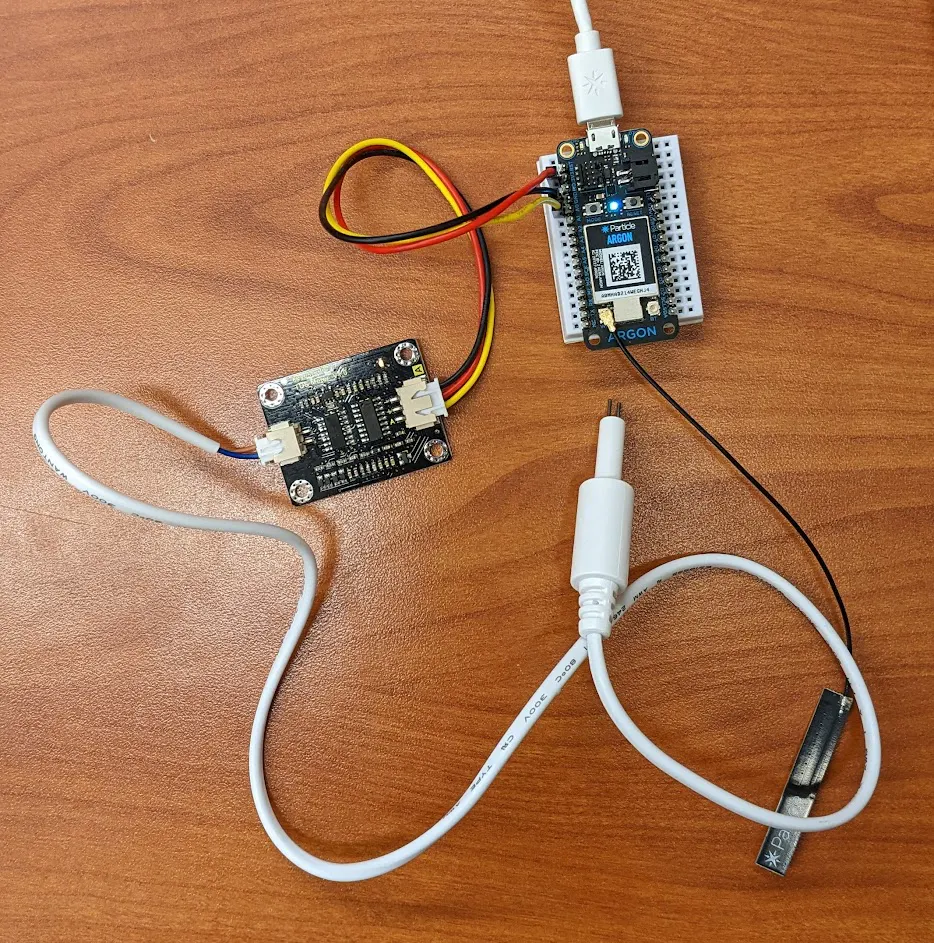### Temp. vs Time Chart

This is the chart acquired from measuring bottled water at room temperature for 30 seconds with the temperature sensor part of our project.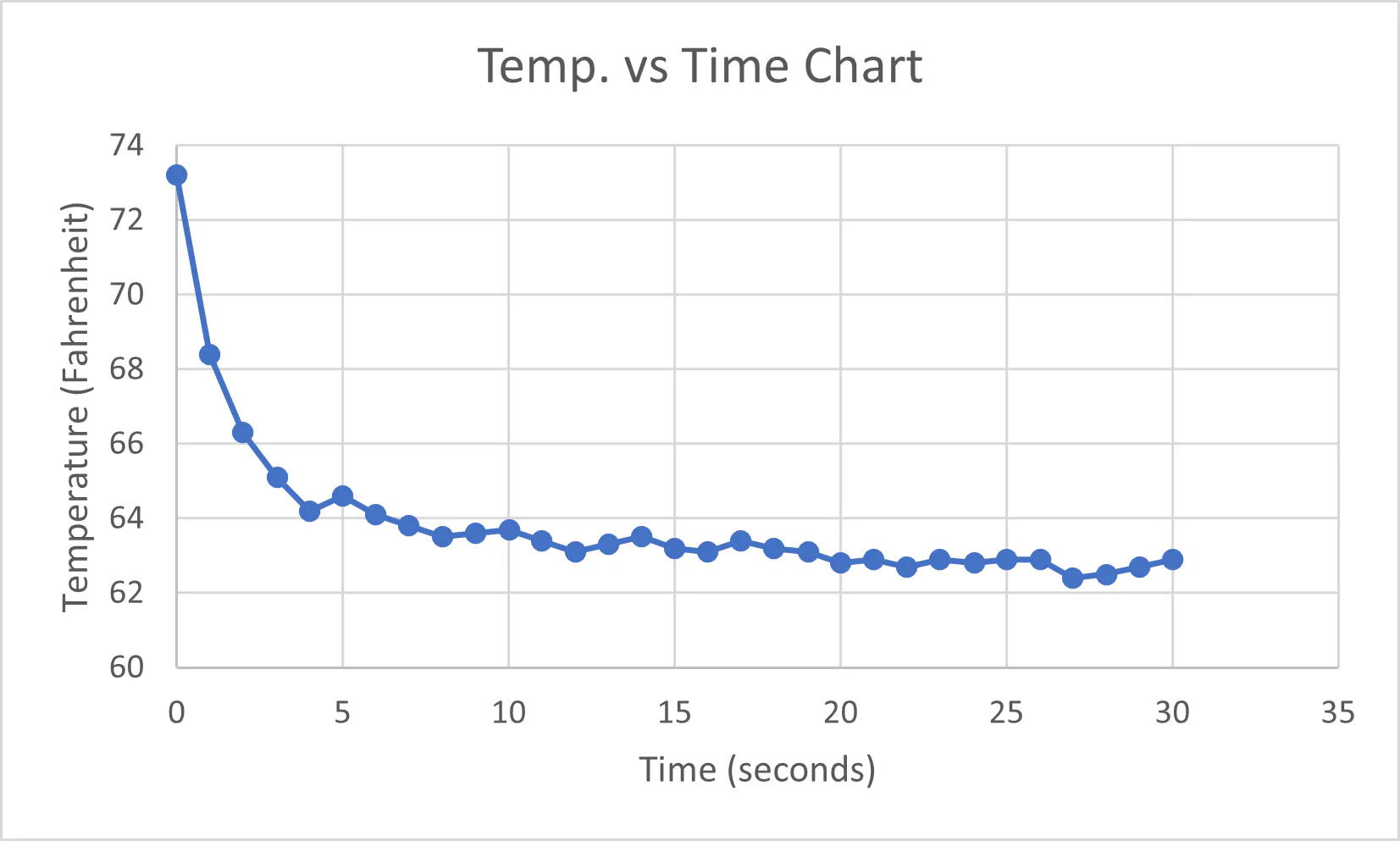### TDS Sensor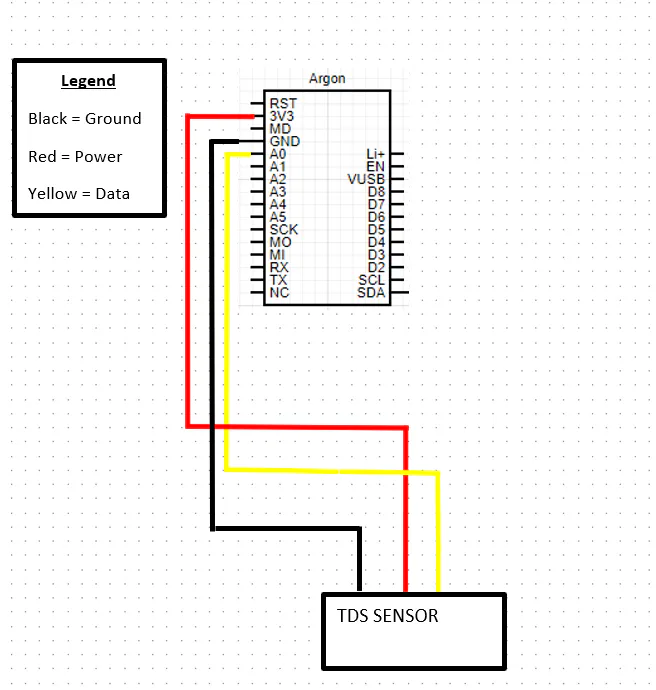### Temperature and pH Circuits

This is both the temperature and pH sensors wired up to the particle Argons via a breadboard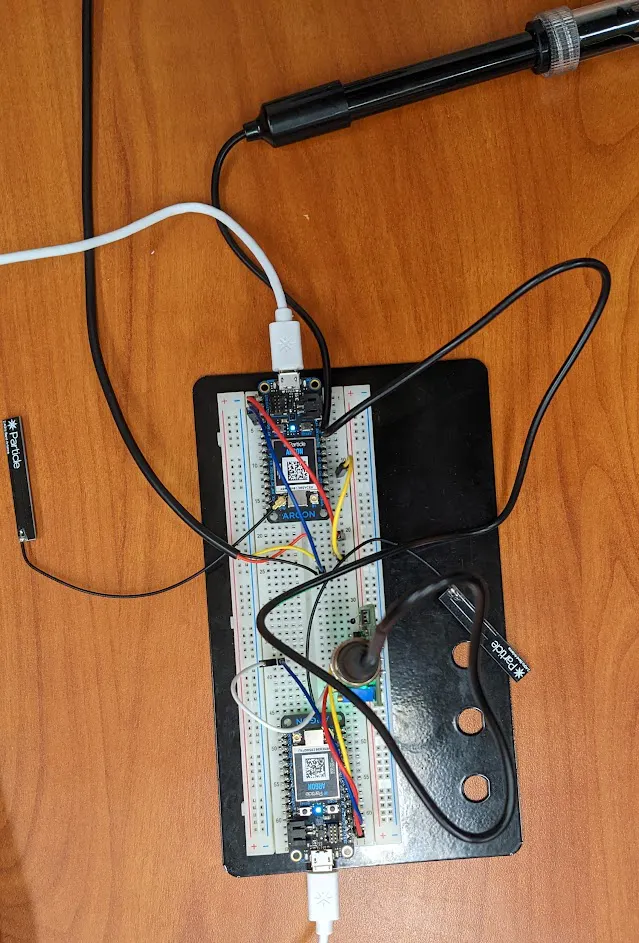## Code

### Gravity TDS Sensor

C/C++
Code for the TDS Sensor
```#define TdsSensorPin A1
#define VREF 3.3      // analog reference voltage(Volt) of the ADC
#define SCOUNT  30           // sum of sample point
int analogBuffer[SCOUNT];    // store the analog value in the array, read from ADC
int analogBufferTemp[SCOUNT];
int analogBufferIndex = 0,copyIndex = 0;
float averageVoltage = 0,tdsValue = 0,temperature = 25;

void setup()
{
Serial.begin(115200);
pinMode(TdsSensorPin,INPUT);
}

void loop()
{
static unsigned long analogSampleTimepoint = millis();
if(millis()-analogSampleTimepoint > 40U)     //every 40 milliseconds,read the analog value from the ADC
{
analogSampleTimepoint = millis();
analogBuffer[analogBufferIndex] = analogRead(TdsSensorPin);    //read the analog value and store into the buffer
analogBufferIndex++;
if(analogBufferIndex == SCOUNT)
analogBufferIndex = 0;
}
static unsigned long printTimepoint = millis();
if(millis()-printTimepoint > 800U)
{
printTimepoint = millis();
for(copyIndex=0;copyIndex<SCOUNT;copyIndex++)
analogBufferTemp[copyIndex]= analogBuffer[copyIndex];
averageVoltage = getMedianNum(analogBufferTemp,SCOUNT) * (float)VREF / 1024.0; // read the analog value more stable by the median filtering algorithm, and convert to voltage value
float compensationCoefficient=1.0+0.02*(temperature-25.0);    //temperature compensation formula: fFinalResult(25^C) = fFinalResult(current)/(1.0+0.02*(fTP-25.0));
float compensationVolatge=averageVoltage/compensationCoefficient;  //temperature compensation
tdsValue=(133.42*compensationVolatge*compensationVolatge*compensationVolatge - 255.86*compensationVolatge*compensationVolatge + 857.39*compensationVolatge)*0.5;
Particle.publish("TDS Value:");
Serial.print(tdsValue,0);
Serial.println("ppm");
}
}
int getMedianNum(int bArray[], int iFilterLen)
{
int bTab[iFilterLen];
for (byte i = 0; i<iFilterLen; i++)
bTab[i] = bArray[i];
int i, j, bTemp;
for (j = 0; j < iFilterLen - 1; j++)
{
for (i = 0; i < iFilterLen - j - 1; i++)
{
if (bTab[i] > bTab[i + 1])
{
bTemp = bTab[i];
bTab[i] = bTab[i + 1];
bTab[i + 1] = bTemp;
}
}
}
if ((iFilterLen & 1) > 0)
bTemp = bTab[(iFilterLen - 1) / 2];
else
bTemp = (bTab[iFilterLen / 2] + bTab[iFilterLen / 2 - 1]) / 2;
return bTemp;
}
```

### pH sensor code

C/C++
Code for the pH Sensor
```int pH_Value;
float Voltage;

void setup()
{
Serial.begin(9600);
pinMode(pH_Value, INPUT);
}

void loop()
{
Voltage = pH_Value * (5.0 / 1023.0);
Serial.println(Voltage);
delay(500);
}
```

### Temperature sensor code

C/C++
Code for temperature collection from the DS18B20 sensor
```#include <OneWire.h>
#include <DS18.h>
DS18 sensor(D4);

char temp;
float current_temp = 0;
float previous_temp = 0;

void setup() {
Particle.variable("Temerature", temp);
}

void loop() {
tempSensor();
delay(1000);
}

void tempSensor()
{
{
current_temp = sensor.celsius();
Serial.println(current_temp);
if (abs(current_temp - previous_temp) >=1)
{
snprintf(temp, sizeof(temp), "Temperature %0.2f", current_temp);
Particle.publish("Temperautre", String(sensor.celsius()),PRIVATE);
previous_temp = current_temp;
}
else
{
Serial.println("Change in Temperature is <1*C");
}
}
}
```

## Credits

### Keagan Michael

1 project • 0 followers

### Christian Del Angel

0 projects • 0 followers

### Arlen Smith

0 projects • 0 followers